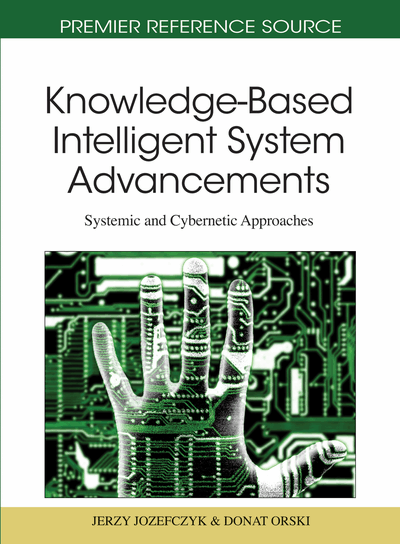# Nonlinear Adaptive Ship Control Synthesis In The Case Of Model Uncertainty

Zenon Zwierzewicz (Szczecin Maritime University, Poland)
DOI: 10.4018/978-1-61692-811-7.ch018
Available
\$37.50
No Current Special Offers

## Abstract

This chapter covers the concerns with a problem of adaptive ship control synthesis in the case of substantially limited knowledge of the plant model. In fact we have at our disposal only its highly general structure in the form of Norrbin’s-like representation with unknown nonlinearities. Two tasks of ship control are considered. The first task is concerning the ship course-keeping system design while the second refers to the path-following system. Two different approaches to the control synthesis problem are considered. One is based on an adaptive feedback linearization technique, while the second refers to the backstepping method where the tuning of unknown parameters is also taken into account. It has been demonstrated that the controllers thereby obtained enable on-line learning of unknown model characteristics, having at the same time the performance comparable to the case of fully known model parameters. The system’s performance assessment for the each case has been tested via Matlab/Simulink simulations.
Chapter Preview
Top

## Introduction

A common problem of engineering practice is to cope with mathematical models of objects with only partly known structure. The model may e.g. involve some unknown (linear or nonlinear) functions that depend on the kind of object (of a given class to which the model refers) and/or of its operational conditions. As an example we take a general model of a single-input-single-output (SISO) systemy(n) = f(x) +g(x)u(1) that describes a wide class of objects. x = [x, x,L, x(n-1)]T denotes here a system state, u is a control input, y = x an output while the functions f and g are unknown or may be estimated with a considerable inaccuracy. More general classes of nonlinear control systems, not necessarily originally of the form (1) can be transformed into this structure, see e.g., Fabri & Kadrikamanathan (2001) or Spooner & Passino (1996) for details. One of the known methods of tracking control synthesis, in a case when we have a rough estimate of the above model functions, is a sliding mode control law (Slotine & Li, 1991). The alternative is to use adaptation, which offers a more subtle policy but requires a more advanced theory.

The problem of adaptive control of nonlinear systems belongs to the class of problems which suffers a lack of sufficiently general and coherent theory. In this chapter two methods of adaptive, nonlinear control synthesis are considered via examples of ship control: course-keeping as well as the path-following problem. The main feature of the presented approach is that we have very limited knowledge of the plant model that forms a basis for the control synthesis. It is assumed that nonlinear vessel dynamics is represented by Norrbin’s-like ship model structure with unknown nonlinearities. The unknown model nonlinearities are assumed to be linear combinations of some known model related basis functions (Zwierzewicz, 2007) i.e. some elementary knowledge of the model is assumed. Following the well-known Norrbin model structure (as a specific case of general system structure (1)) which results from experimental studies, the adopted nonlinearity approximator has a form of polynomial with unknown parameters. Generally this assumption may be substantially relaxed via applying, as the basis functions, some sort of known approximators (Fabri & Kadrikamanathan, 2001; Tzirkel-Hancock & Fallside, 1992). As an example one may adopt a neuro-approximator with Gaussian radial basis functions (Sanner & Slotine, 1992). Systems of this sort are referred to as functional adaptive (Fabri & Kadrikamanathan, 2001) and represent a new branch of intelligent control systems.

As the model parameters are difficult for identification the proposed approach assumes a direct adaptation i.e. that without the requirement of model parameter estimation. The first of the proposed methods is based on cancellation of nonlinear terms i.e. feedback linearization technique is mildly used here. The second approach uses the recently popularized method of backstepping (Krstic at al., 1995). In both cases, for the tuning of unknown model parameters, the Lyapunov function technique is exploited. The main drawback of feedback linearization in relation to the backstepping method is that the first leads to the cancellation of all system nonlinearities irrespective of their positive system influence while the backstepping is much more flexible in this respect.

## Complete Chapter List

Search this Book:
Reset